Browse by Topic
Related Topics

# Exploring Maxwell’s Equations with Mathematica 9

I love Maxwell’s equations. As a freshman in college, while pondering whether to major in physics, computer science, or music, it was the beauty of these equations and the physical predictions that can be elegantly extracted from them that made me decide in favor of physics. On a more universal level, the hints in Maxwell’s equations led Einstein to write Zur Elektrodynamik bewegter Körper (“On the Electrodynamics of Moving Bodies”), more commonly known as Einstein’s first paper on the theory of relativity. The quantum version of the equations, quantum electrodynamics (QED), remains our most successful physical theory, with predictions verified to 12 decimal places. There are many reasons to love Maxwell’s equations. And with Mathematica 9’s new vector analysis functionality, exploring them has never been easier.

So what exactly are Maxwell’s equations? They are a set of four partial differential equations that describe how electric and magnetic fields respond to charges, currents, and each other. In 1861, James Clerk Maxwell corrected and combined four disparate equations that had been known in one form or another in order to create a comprehensive theory of electromagnetism. In natural Lorentz-Heaviside units, they take the following form.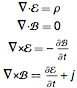In the above, ℰ is the electric field, ℬ is the magnetic field, ρ is the charge density, and j is the current density. The operation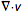is called the divergence of v and is a measure of whether the field in a region is pointing away from a point (a positive number), in toward the point (a negative number), or uniformly across it (zero). Finally,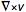is the curl of v and is a measure of how quickly and in what direction the field is swirling about a particular point. Thus, while Maxwell’s equations look complicated—and have many interesting mathematical properties—they can be summarized as follows:

1) Electric fields point away from positive charges and toward negative charges.

2) Magnetic fields never point in or out of a single location, but only point uniformly in the same direction or form closed loops.

3) Electric fields swirl when there is a magnetic field changing in time.

4) Magnetic fields swirl when there is a time-varying electric field or when an electric current is flowing.

Perhaps the most famous solution of Maxwell’s equations is the Coulomb field, which is the electric field and magnetic field of a stationary point with charge q. In spherical coordinate {r,θ,φ} these have the form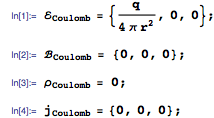We can verify that these are in fact a solution to Maxwell’s equation using the new Div and Curl functions. These take the field to be differentiated, the coordinates, and (optionally) a coordinate system. Since these solutions are expressed in spherical coordinates, the syntax is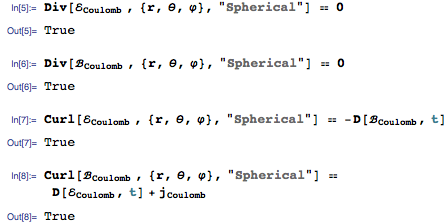Using the new function TransformedField, we can convert the electric field to Cartesian coordinates {x,y,z}.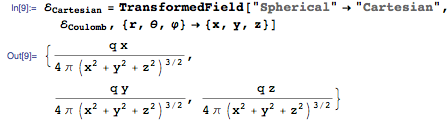There is no need to convert the other fields, since they are all zero and therefore will remain zero in all coordinate systems. Still, we can verify that &#8496Cartesian satisfies Maxwell’s equations in the new coordinate systems.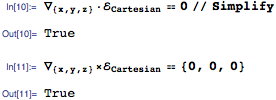Here is a visualization of the electric field in which the charge has been set to 4π. Since this charge is positive, the field is pointing away from the charge at the center. Also, the magnitude of the field decreases rapidly with distance from the center because it is proportional to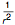.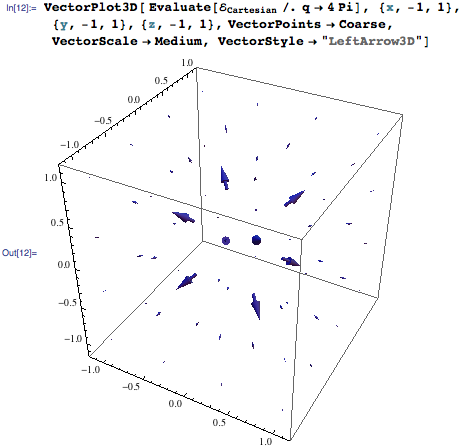Mathematica supports many more coordinate systems than just the basic spherical and Cartesian coordinates. All of them can be found using the function CoordinateChartData. In three dimensions, 14 coordinate systems are supported.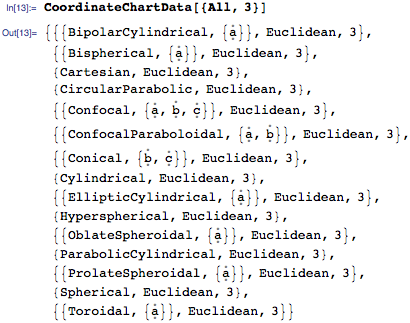One of the most important discoveries Maxwell made was that electric and magnetic fields can form wave configurations which travel from one place to another. The discovery of electromagnetic waves has led to radio, television, radar, and countless other technologies. The derivation is as elegant as it is short. Assuming we are in vacuum (ρ==0 and j==0), we take the curl of both sides of the third Maxwell equation, yielding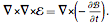Using the following vector identity on the left-hand side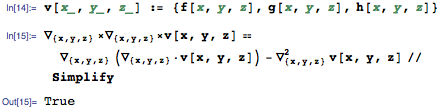and interchanging the order of operations and substituting in the fourth Maxwell equation on the left-hand side yields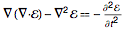But by the first Maxwell equation, given the vacuum condition, the first term in the equation is also zero. Rearranging produces the famed wave equation for the electric field.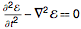Performing a similar analysis of the fourth Maxwell equation produces the same wave equation of ℬ.

One of the simplest solutions to these wave equations is the plane-wave solution given by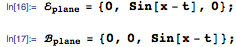This solution represents a uniform beam of light traveling in a single direction. Of course, the solution obeys Maxwell’s equations.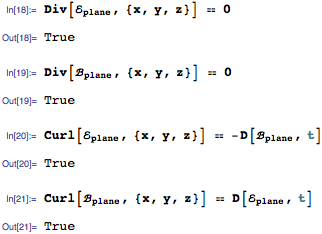It also obeys the specific wave equations derived above.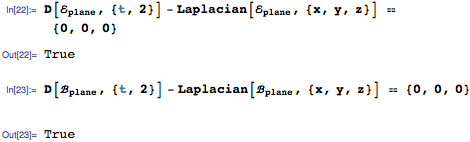Plane wave solutions model electromagnetic waves that are far from the source, as compared with the wavelength of the wave or the size of the source, traveling over relatively short distances. If we plot intensity ℐ = |ℰxℬ| as a function of position and let time vary, we see a wave pattern moving to the right.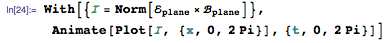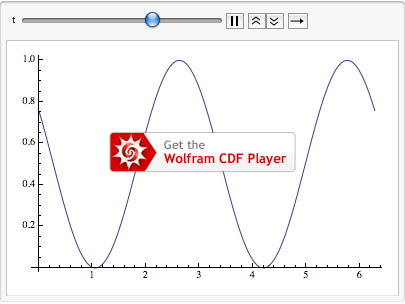Another simple but important approximate solution to Maxwell’s equation is the dipole radiation field, given in spherical coordinates by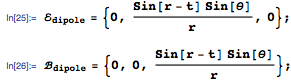As these are approximate solutions, they do not exactly satisfy Maxwell’s equations. They do satisfy the second and third equations—the so-called homogeneous equations that have no charges or currents in them.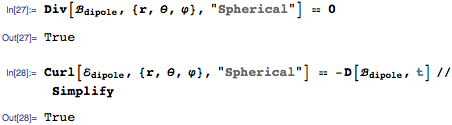However, because terms containinghave been dropped from the solutions, ℰdipole and ℬdipole only satisfy the other Maxwell equations if terms proportional toare ignored.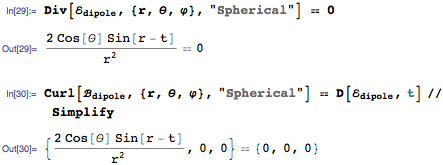These approximate solutions are useful because they model the electric field far from a radiating source, for example a radio transmitter. Since r is assumed to be large, the terms proportional toare negligible compared with the terms that are kept. Again, we use the intensity to visualize the field. In spherical coordinates, we have the intensity as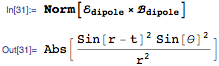Since the intensity does not depend on azimuthal angle φ, it does not matter which direction in the xy plane we consider. We therefore convert to Cartesian coordinates and restrict to the xz plane (y==0).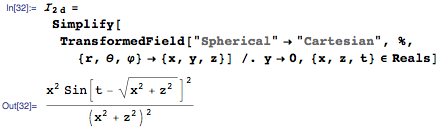We can now make a density plot in the xz plane. Bright color corresponds to high intensity and dark color to low intensity. You can see that as the distance from the source increases, the intensity falls off. Moreover, as time increases, new crests of high intensity travel outward from the center and slowly decrease in intensity.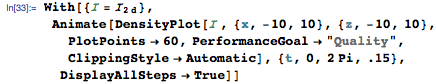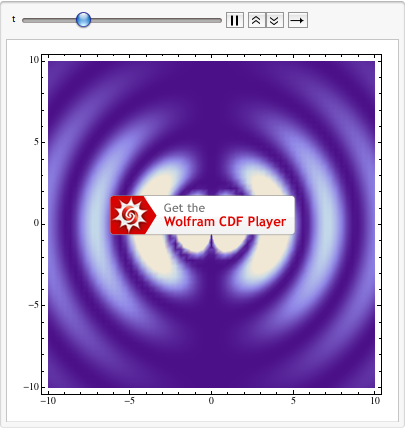The solutions discussed above are some of the simplest known solutions of Maxwell’s equations. There is no completely general solution in terms of known functions, so a variety of different techniques for solving the equations in different applications have been developed. In the case of time-independent fields, it is common to use potential functions. For example, we can write ℰ = -∇V (which is always possible in this case, since ∇xℰ == 0 by the third Maxwell equation), and then focus on the first equation to get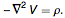This is Poisson’s equation, or, for ρ == 0, Laplace’s equation. In cylindrical coordinates, it takes the form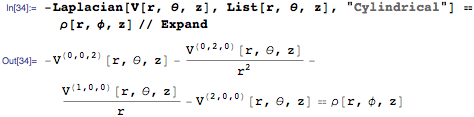In the case of cylindrical symmetry, when V and ρ depend only on r, DSolve can return an answer for any density function.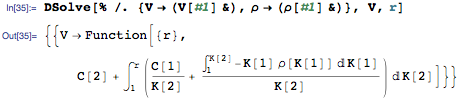For time-dependent situations, it is common to use Green’s functions, which are essentially solutions for a point particle in a form that can be integrated to give the solution for an arbitrary charge distribution. For more complicated situations, it may be necessary to use numerical methods as embodied by NDSolve. But whether exploring Maxwell’s equations symbolically, numerically, or visually, Mathematica has the tools for the job.